Tuesday 27th October 2020CBSE Guess > Papers > Important Questions > Class XII > 2009 > Maths > Mathematics By Anil Kumar Tondak

CBSE CLASS XII

Determinants

Q. 1. Prove that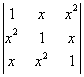= (1- x3)2

Q. 2. Find the equation of the line joining A(1,3) and B(0,0) using determinants and find  if D (K, 0) is a point such that area of a triangle ABD is 3 square units.

Q. 3. If

A =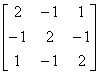Verify that A3 – 6A2 + 9A – 4I = 0 and hence find A-1

Q. 4. Prove that :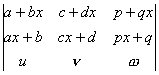= (1 – x2)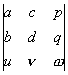Q. 5. Solve by matrix method:
2x + y + z = 1
= 3/2
5z = 9

Q. 6. Prove that :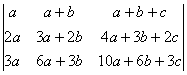= a3

Q. 7. Prove that :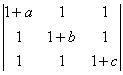= abc + bc + ca + ab.

Q. 8. Solve :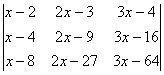= 0

Q. 9. Using determinants, find the area of the triangle whose vertices are (1, 4), (2, 3), (-5, 3). Are the given points collinear.

Q. 10. If the points (a1, b1),  (a2, b2) and (a1 + a2 b1 + b2) are collinear, Show that a1b2 = a2b1.

Q. 11. If a, b, c are all positive and are pth , qth and rth terms of G.P., then show that
D =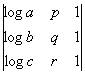= 0

Q. 12. If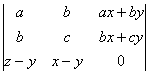= 0, then Prove that
a, b, c are in G.P  or  x, y, z are in G.P

Prepared By Anil Kumar Tondak
M.Sc.(Maths), M.Ed., M.A.(Eco),M.B.A.(Mkt)
Mob No. 9811363962

 Maths